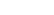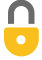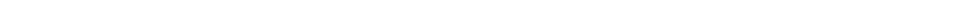Problem 17
Q

# When a 290-g piece of iron at $180 ^\circ \textrm{C}$ is placed in a 95-g aluminum calorimeter cup containing 250 g of glycerin at $10 ^\circ \textrm{C}$, the final temperature is observed to be $38 ^\circ \textrm{C}$. Estimate the specific heat of glycerin.A
$2300 \textrm{ J/kg C}^\circ$In order to watch this solution you need to have a subscription.VIDEO TRANSCRIPT

This is Giancoli Answers with Mr. Dychko. When this piece of iron is placed into a calorimeter made of aluminum containing some glycerin, the iron is going to lose some heat and the glycerin and calorimeter cup are both gonna gain some heat. So, total heat gained for the glycerin and the cup is gonna be mass of the cup times the specific heat of the cup times its change in temperature, final temperature minus the initial temperature of the cup, plus the mass of the glycerin times the specific heat of glycerin times the final temperature minus the initial temperature of the glycerin. Notice the final temperature does not have a subscript because they all have all 3 things reach the same final temperature. I don't think, I don't suppose there needs to be a subscript to distinguish the calorimeter from the glycerin initial temperatures either since they start at the same initial temperature, but doesn't hurt to put it there anyway. And that's gonna equal, this heat gain is gonna be equal to the heat loss by the iron which is initial temperature of the iron minus its final temperature times the mass of the iron times the specific heat of iron. And we can solve all this for a specific heat of glycerin by moving this term to the right hand side which makes it a minus mc cc Tf minus Tic. And then also dividing by mass of glycerin and dividing both sides by mass or temperature final minus temperature initial glycerin which is what you see in the denominator here. And that solves for specific heat of glycerin. So, that's going to be 0.29 kilograms mass of the piece of iron placed into the calorimeter times 450 joules per kilogram Celsius degree, specific heat of iron, times 180 degrees Celsius, initial temperature of the iron minus its 38 degrees Celsius, final temperature, minus 0.095 kilograms, mass of the calorimeter cup times 900 joules per kilogram Celsius degree, specific heat of aluminum, which is what the calorimeter cup is made of, times 38 degrees Celsius minus the calorimeter's initial temperature of 10 degrees Celsius divided by 0.5 kilograms mass of glycerin within the calorimeter times the 38 degrees, the final temperature, minus 10 degrees Celsius, initial temperature of glycerin. And this gives about 2,300 joules per kilogram Celsius degree is the specific heat of glycerin.

Giancoli Answers, including solutions and videos, is copyright © 2009-2023 Shaun Dychko, Vancouver, BC, Canada. Giancoli Answers is not affiliated with the textbook publisher. Book covers, titles, and author names appear for reference purposes only and are the property of their respective owners. Giancoli Answers is your best source for the 7th and 6th edition Giancoli physics solutions.